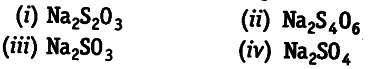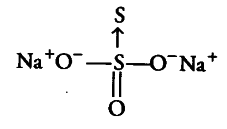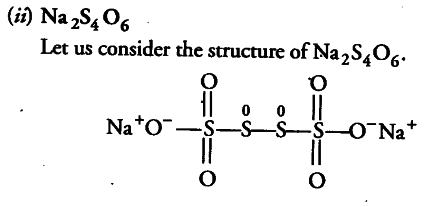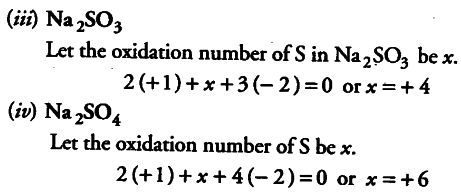# Calculate the oxidation number of each sulphur

Calculate the oxidation number of each sulphur atom in the following compounds(i) Na_{2}S_{2}O_{3} Let us consider the structure of Na_{2}S_{2}O_{3}.There is a coordinate bond between two sulphur atoms. The oxidation number acceptor S atom is — 2. Let the oxidation number of other S atom be x.
. 2 (+ 1)+ 3x (— 2) +x+ 1 (— 2) =0
x = +6
Therefore, the two sulphur atoms in Na_{2}S_{2}O_{3}. have -2 and +6 oxidation state.In this structure, two central sulphur atoms have zero oxidation number because electron pair forming the S—S bond remain in the centre. Let the oxidation number of (remaining S atoms) S atom be x.
2(+ l)+6(-2)+2x + 2(0)=0

2 —12+2x=0 or x = + 5
Therefore, the two central S atoms have zero oxidation state and two terminal S atoms have + 5 oxidation state each.